# What should be the spring constant k of a spring designed to bring a 1420 kg...

What should be the spring constant k of a spring designed to bring a 1420 kg car to rest from a speed of 88 km/h so that the occupants undergo a maximum acceleration of 5.0 g?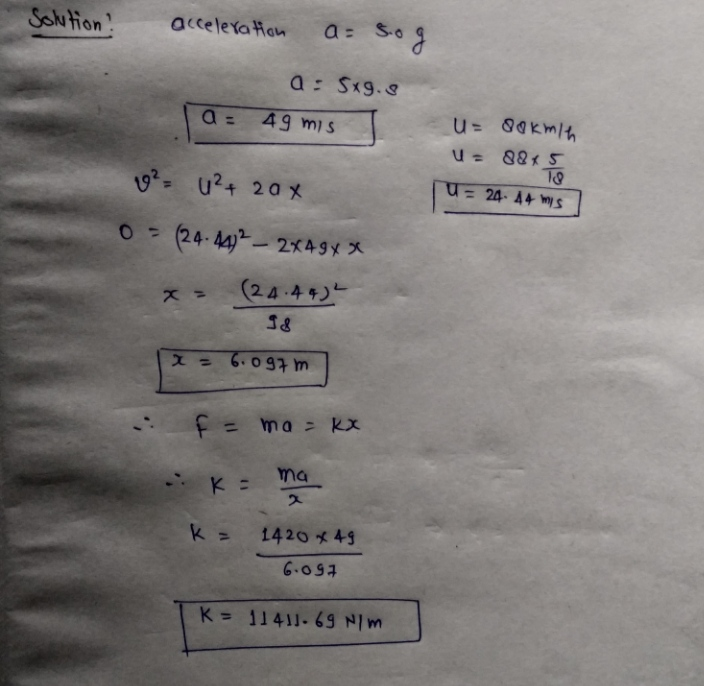#### Earn Coin

Coins can be redeemed for fabulous gifts.

Similar Homework Help Questions
• ### What should be the spring constant k of a spring designed to bring a 1200 kg car to rest from a speed of 101 km/h so that the occupants undergo a maximum acceleration of 5.0 g

What should be the spring constant k of a spring designed to bring a 1200 kg car to rest from a speed of 101 km/h so that the occupants undergo a maximum acceleration of 5.0 g?

• ### A 2 kg mass is attached to a spring and placed on a frictionless horizontal surface....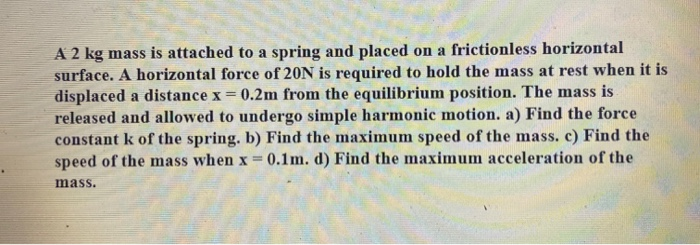A 2 kg mass is attached to a spring and placed on a frictionless horizontal surface. A horizontal force of 20N is required to hold the mass at rest when it is displaced a distance x = 0.2m from the equilibrium position. The mass is released and allowed to undergo simple harmonic motion. a) Find the force constant k of the spring. b) Find the maximum speed of the mass. c) Find the speed of the mass when x =...

• ### A 0.40-kg mass is attached to a spring with a force constant of k = 207...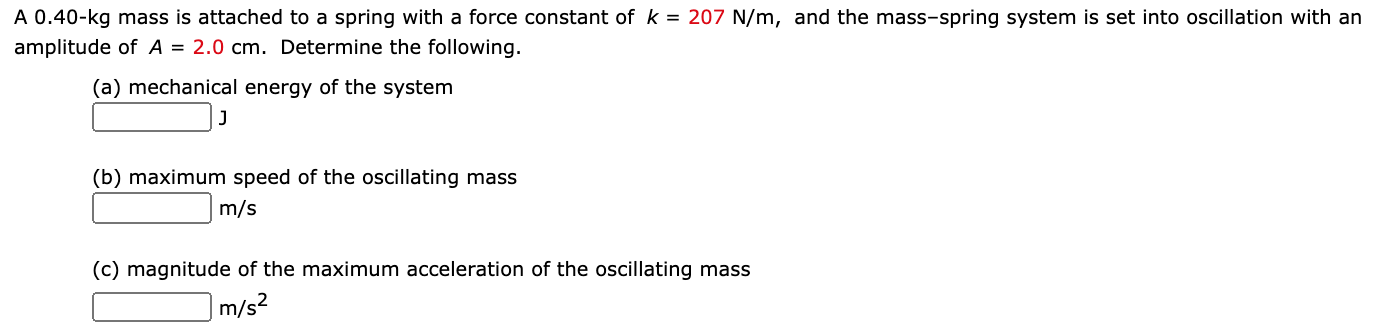A 0.40-kg mass is attached to a spring with a force constant of k = 207 N/m, and the mass–spring system is set into oscillation with an amplitude of A = 2.0 cm. Determine the following. (a) mechanical energy of the system _____ J (b) maximum speed of the oscillating mass _____ m/s (c) magnitude of the maximum acceleration of the oscillating mass _____ m/s2 A 0.40-kg mass is attached to a spring with a force constant of k =...

• ### A device is being designed that can be modeled as a mass-spring system. The mass-spring constant ...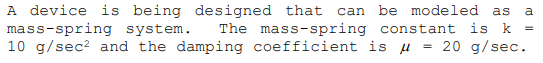A device is being designed that can be modeled as a mass-spring system. The mass-spring constant is k - 10 g/sec2 and the damping coefficient is μ 20 g/sec. a. Now the mass is pulled down 5 cm from rest and given an upward velocity of 10 cm/sec. Determine the IVP describing the motion of the mass b. Solve the resulting DE from part a Sketch the graph of the motion. d. Find the maximum displacement of the mass once...

• ### A 78-kg man stands on a spring scale in an elevator

A 78-kg man stands on a spring scale in an elevator. Starting from rest, the elevator ascends, attaining its maximum speed of 1.2 m/s in 0.74 s. The elevator travels with this constant speed for 5.0 s, undergoes a uniform negative acceleration for 1.8 s, and then comes to rest. What does the spring scale register in each of the following time intervals? (a) before the elevator starts to move (b) during the first 0.74 s of the elevator's ascent...

• ### * Amass of 2.0 kg is connected to a spring with a spring constant of 5.0...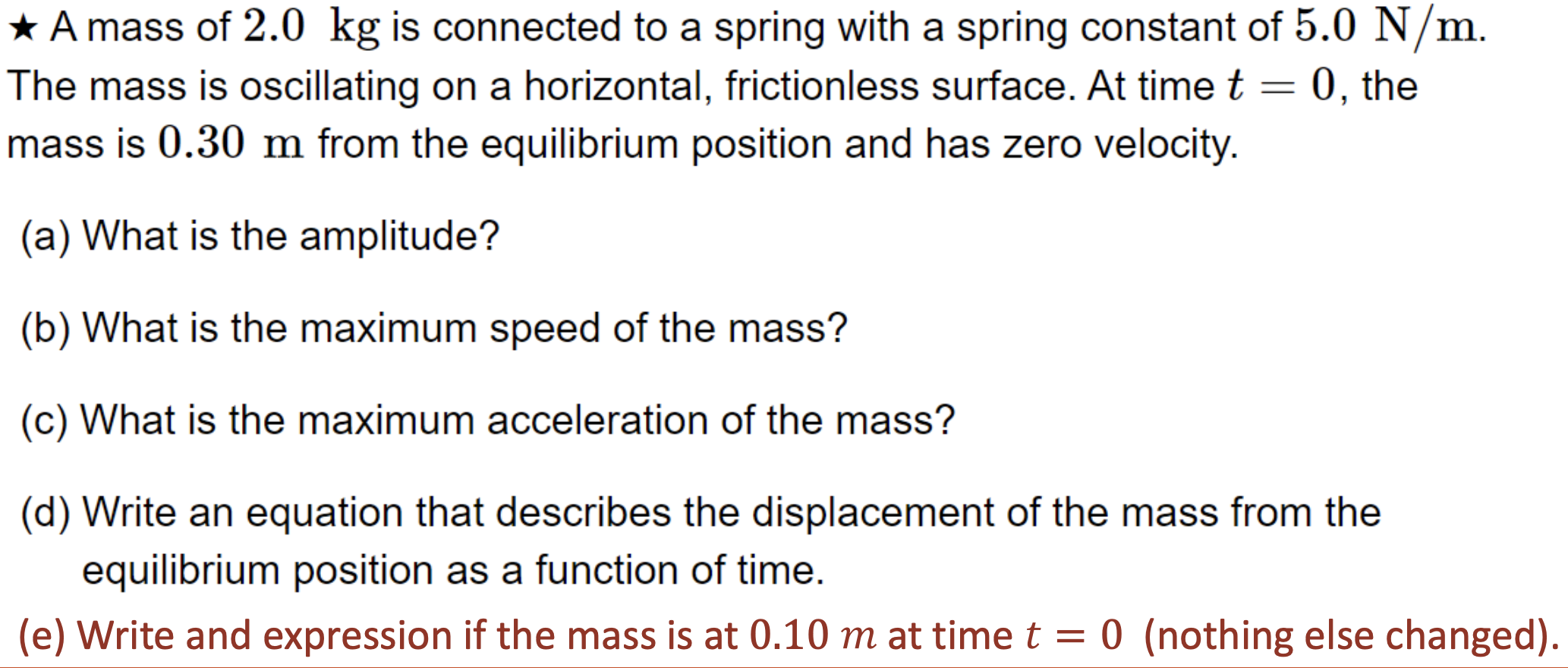* Amass of 2.0 kg is connected to a spring with a spring constant of 5.0 N/m. The mass is oscillating on a horizontal, frictionless surface. At time t = 0, the mass is 0.30 m from the equilibrium position and has zero velocity. (a) What is the amplitude? (b) What is the maximum speed of the mass? (c) What is the maximum acceleration of the mass? (d) Write an equation that describes the displacement of the mass from the...

• ### A 0.64 kg mass is attached to a light spring with a force constant of 23.9...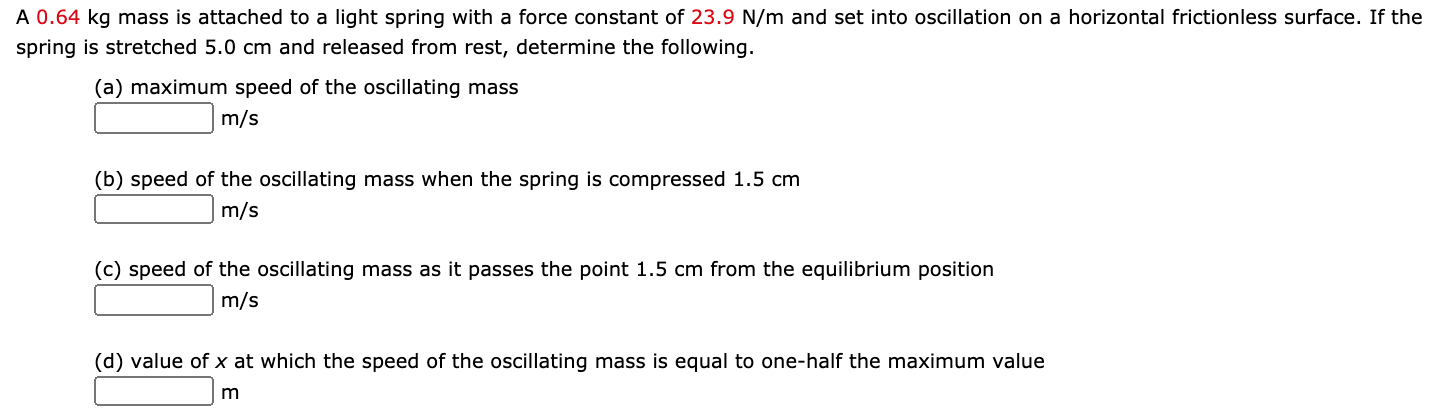A 0.64 kg mass is attached to a light spring with a force constant of 23.9 N/m and set into oscillation on a horizontal frictionless surface. If the spring is stretched 5.0 cm and released from rest, determine the following. (a) maximum speed of the oscillating mass _____ m/s (b) speed of the oscillating mass when the spring is compressed 1.5 cm _____ m/s (c) speed of the oscillating mass as it passes the point 1.5 cm from the equilibrium...

• ### A 0.40-kg mass is attached to a spring with a force constant of k = 337...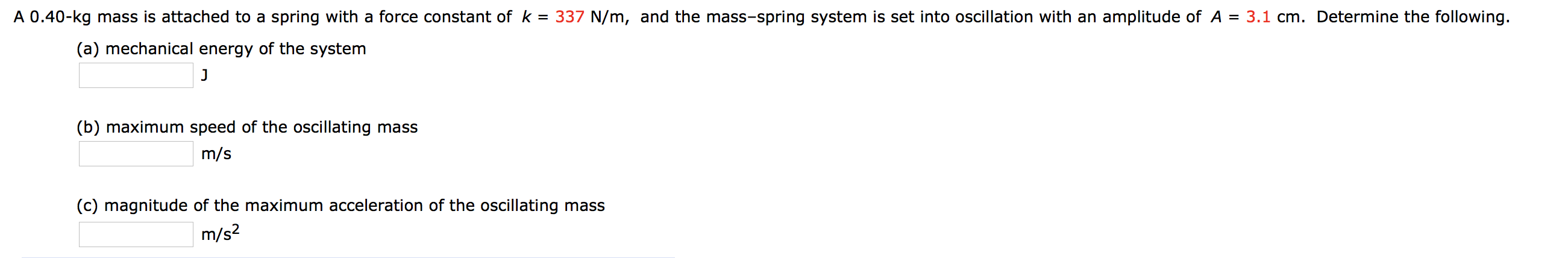A 0.40-kg mass is attached to a spring with a force constant of k = 337 N/m, and the mass-spring system is set into oscillation with an amplitude of A = 3.1 cm. Determine the following. (a) mechanical energy of the system (b) maximum speed of the oscillating mass m/s (c) magnitude of the maximum acceleration of the oscillating mass m/s2

• ### A 2.5-kg object attached to an ideal spring with a force constant (spring constant) of 15...

A 2.5-kg object attached to an ideal spring with a force constant (spring constant) of 15 N/m oscillates on a horizontal, frictionless track. At time t = 0.00 s, the cart is released from rest at position x = 8 cm from the equilibrium position. (a) What is the frequency of the oscillations of the object? (b) Determine the maximum speed of the cart. (c) Find the maximum acceleration of the mass (d) How much total energy does this oscillating...

• ### A 0.40-kg mass is attached to a spring with a force constant of k = 337...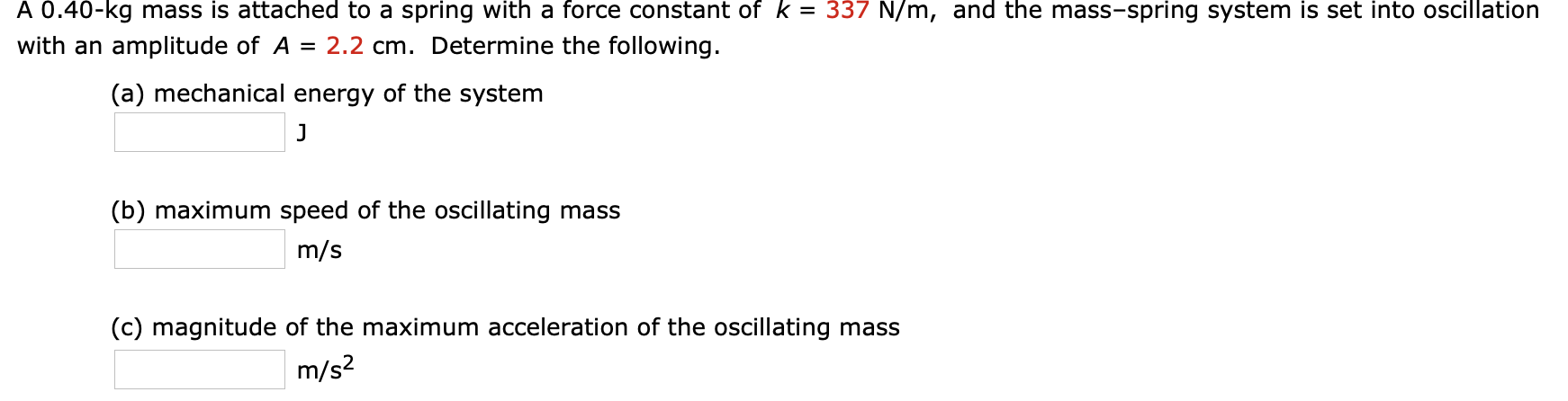A 0.40-kg mass is attached to a spring with a force constant of k = 337 N/m, and the mass-spring system is set into oscillation with an amplitude of A = 2.2 cm. Determine the following. (a) mechanical energy of the system J (b) maximum speed of the oscillating mass m/s (c) magnitude of the maximum acceleration of the oscillating mass m/s2